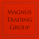2161 views
A CHANGE of background color indicates a trade opportunity :
Purple background = SELL

The bullish signals seem to be better than the bearish ones.

Based on the "Willy" indicator.
Don't change the parameters unless you fully understand the consequences.

Use with brains and caution :)
```study(title="MAGNUS™", shorttitle="MAGNUS™")

length = input(21, minval=1)
upper = highest(length)
lower = lowest(length)

out = 100 * (close - upper) / (upper - lower)
src = out, len = input(54, minval=1, title="MA Short Length")
lenm2 = input(13, minval=1, title="MA Long Length")
len0 = input(8, minval=1, title="Willy Smoothing")

m0 = ema(out, len0)
m1 = ema(out, len)
m2 = sma(out,lenm2)
col = m0>m1?aqua:fuchsia

plot(out, color = green)
plot(m0, color = white)
plot(m2, color = red)
plot(m1, title="EMA", color=orange)
band1 = hline(-20)
band0 = hline(-80)
fill(band1, band0)
bgcolor(col,transp=92)```